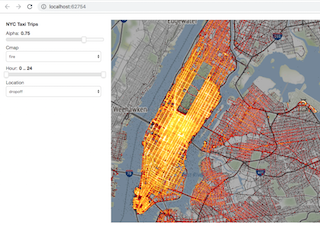# Dashboard¶## NYC Taxi data with Datashader and Panel¶

The NYC Taxi trips dataset is a well-studied data science example. Here we show how to build a simple dashboard for exploring 10 million taxi trips in a Jupyter notebook using Datashader, then deploying it as a standalone dashboard using Panel.

Running the dashboard requires having a live Python process running (not just a static webpage or anaconda.org notebook viewer). Before you run the notebook or server, you'll need to set up a conda environment and run conda install -c pyviz datashader panel geoviews, and you'll need to get the data file.

In :
import holoviews as hv, param, dask.dataframe as dd
import panel as pn

from colorcet import cm
from holoviews.element.tiles import StamenTerrain

hv.extension('bokeh', logo=False)

usecols = ['dropoff_x','dropoff_y','pickup_x','pickup_y','dropoff_hour','pickup_hour','passenger_count']
opts = dict(width=1000,height=600,xaxis=None,yaxis=None,bgcolor='black',show_grid=False)
cmaps = ['fire','bgy','bgyw','bmy','gray','kbc']

class NYCTaxiExplorer(param.Parameterized):
alpha      = param.Magnitude(default=0.75, doc="Alpha value for the map opacity")
cmap       = param.ObjectSelector(cm['fire'], objects={c:cm[c] for c in cmaps})
hour       = param.Range(default=(0, 24), bounds=(0, 24))
location   = param.ObjectSelector(default='dropoff', objects=['dropoff', 'pickup'])

@param.depends('location', 'hour')
def points(self):
points = hv.Points(df, kdims=[self.location+'_x', self.location+'_y'], vdims=['dropoff_hour'])
if self.hour != (0, 24): points = points.select(dropoff_hour=self.hour)
return points

def view(self,**kwargs):
points = hv.DynamicMap(self.points)
tiles = StamenTerrain().apply.opts(alpha=self.param.alpha, **opts)
agg = rasterize(points, x_sampling=1, y_sampling=1, width=600, height=400)